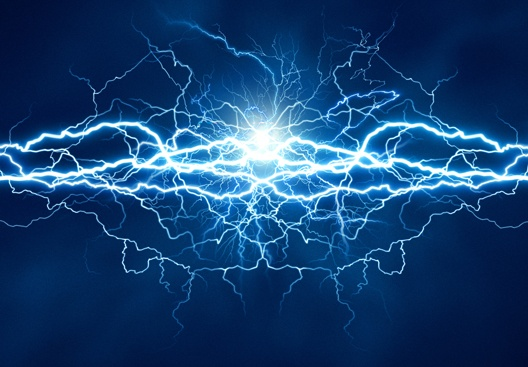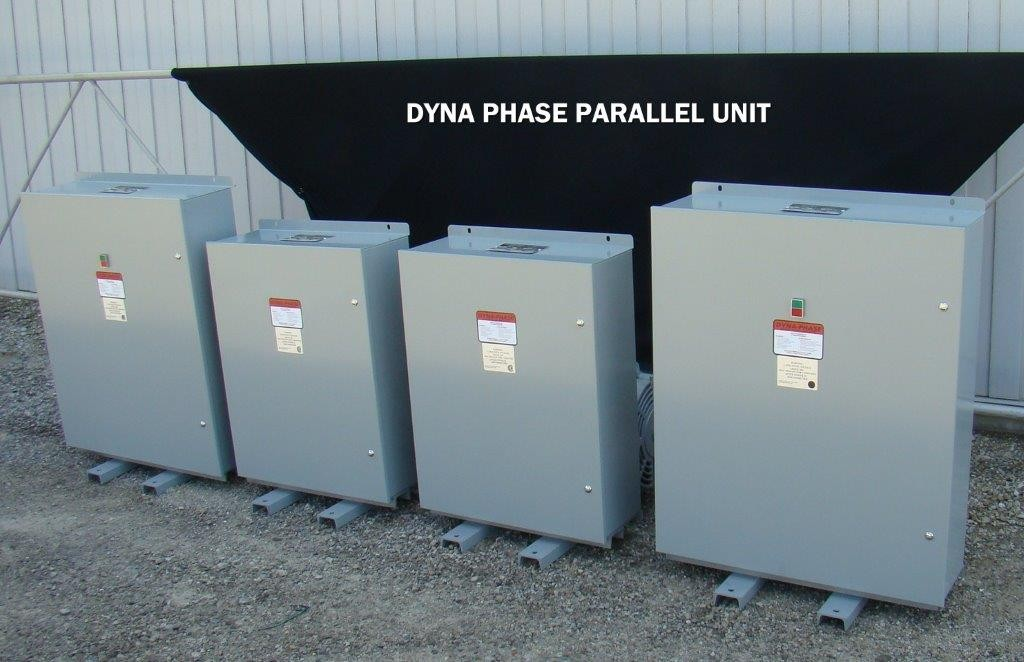# How to Measure a 3 Phase Power Current

When Your Business needs to know what type of Power you have.
Here's how to measure a 3 phase power current.Perhaps the idea of measuring a 3 phase power current sounds intimidating to some of you. After all, a reliance on 3 phase power sources doesn't necessarily indicate an understanding of (or even interest in) the math which lies behind them.

Even so, some of you might be a tad bit curious, on how power is rated.

## So how do you calculate 3 phase power?

While you're certainly welcome to take a peek at Wikipedia's technical entry, we think that you'll find our simple approach to the this task a bit more...suitable for beginners.

That being said, let's hop right into things. Our first order of business is to establish the variables.

## Putting the Variables on the Table

Every good lesson should clearly define the variables at its start, and, needless to say, we want this to be a good lesson. Consequently, we'll be taking this time to briefly touch on watts, apparent power, and power factors.

A watt (W) is a measurement of power. This unit is used to measure the power a circuit takes in. Kilowatts (kW) can also be used to measure this power; one kilowatt is the equivalent of 1000 watts.

Apparent power (VA or volt-ampere) is calculated by finding the product of the voltage and the current; apparent power can also be measured in kilovolt-amperes (kVA). A kVA is equal to 1000 volt-amperes.

The power factor (pf) is the relationship between kilo-volt amperes and kilowatts. It can be represented as:

kW = kVA x pf

Notice that this formula can be algebraically rearranged in order to calculate each component. The power factor, for instance, can be represented as:

pf = kW/kVA

The kilo-volt amperes, on the other hand, can be represented as:

kVA = kW / pf

## Calculating a Single Phase Power Current

Although our ultimate goal is to teach you how to calculate a 3 phase power current, we (and most other people) presume that teaching you how to calculate a single phase power current will lay some important groundwork for what you have at your business now and
what you may be needing in the future.

There are two reasons for our presumption here, the first of which is that calculating a single phase power current is much simpler than calculating a multiphase or three
phase power current.

The second, more important reason has everything to do with the fact that you can actually use the logic and formula for calculating single phase power currents when calculating multiphase power currents.But enough of that talk. Let's get down to business.

Generally speaking, you won't be responsible for calculating all of the values of the variables; some, such as the voltage or power factor, will be supplied. You presumably don't, after all, have access to a voltmeter or any other instrument of that nature.

Having said that, you can easily use the variables that you know the value of to find any unknown values. If, for example, you are given the power factor and wattage, you can make quick work of finding the apparent power.

Remember that the power factor is the relationship between the kilo-volt amperes and kilowatts. This relationship has previously been expressed as:

kW = kVA x pf

If we algebraically rearrange this equation in order to solve for the apparent power (kVA), we get:

kVA = kW/pf

Thus, we can divide our wattage by the power factor in order to find our apparent power.

What, however, do we make of this apparent power?

At this point, we must introduce a new formula that will allow us to calculate the current. Luckily for us, there is a simple one:

Current = kVA (or VA)/voltage

Using this formula, we simply divide the kVA we've calculated by the voltage (which should be given) in order to calculate the current.

## Calculating a 3 Phase Power Current

Now that we've calculated a single phase power current, we can move on to doing the same for 3 phase power currents. Although there is a formula for calculating 3 phase power currents, we'll be teaching you a more intuitive way of completing this task.

Before we get into the math, however, you must understand exactly how a 3 phase system differs from a single phase system.

To put things simply, the crucial difference between the two systems is the voltage; three phase systems have line to line voltage (VLL) and phase voltage (VLN).

The relationship between the line to line voltage and the phase voltage can be written as:

VLN = VLL/sqrt(3)

For our purposes, you don't need to have an in-depth understanding of these two variables. You only need to keep the relationship between them in mind.

You also shouldn't worry about calculating both of them; at least one of them will be given to you.

Using the method we'll be teaching you, the general idea is to convert a 3 phase system into a single phase one.

In order to make this conversion, however, you need to understand that, for our purposes here, a 3 phase system is essentially producing 3 times as many kilowatts as a single phase system; this difference in power produced actually makes it pretty easy to see why some people upgrade to 3 phase power.

The apparent power is also tripled in a 3 phase system.

That said, in order to calculate a 3 phase power current using this method, you'll need to divide your wattage by 3 before plugging the value into this formula:

kVA = kW/pf

You should notice that this is the exact same formula that was used above for single phase systems.

You should then follow this formula up by dividing the kVA by the voltage (your VLN in the case of a 3 phase system) in order to calculate the current.

In this case, however, there is an added step.

Remember that you divided by 3 in order to set up an equation for a single phase system. Because of this division, then, your answer only reflects the output of a single phase.

In order to find the output of the 3 phase system you started with, you need only multiply the current you calculated by 3.

Simple, right?

Well, only if the system is balanced.

Although our calculations assume that a 3 phase system will be balanced, the reality of things is that most systems are not so conveniently balanced. That is to say, each phase doesn't always produce an equal amount of power.

In such cases, you'll need to rely on much more complicated math to get an accurate answer. That math, however, is a bit too complex (polar coordinates and all) to go into detail about here.

### So what do you do? How about more POWER?As it turns out, some sources say that you can take the average of the 3 phases and use that value in your equations. Still, it should be noted that this method is not going to get an exact answer.

Even if you can't calculate the exact answer when dealing with an unbalanced system, though, you've at least figured out (numerically, of course) just what makes 3 phase power that popular kid on the playground that many businesses want on their side.

And who knows? You might even want it on your side one day. Want to inquire more